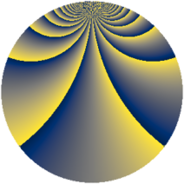# Properties

 Label 912.2.brLevel $912$ Weight $2$ Character orbit 912.br Rep. character $\chi_{912}(221,\cdot)$ Character field $\Q(\zeta_{12})$ Dimension $624$ Newform subspaces $1$ Sturm bound $320$ Trace bound $0$

# Related objects

## Defining parameters

 Level: $$N$$ $$=$$ $$912 = 2^{4} \cdot 3 \cdot 19$$ Weight: $$k$$ $$=$$ $$2$$ Character orbit: $$[\chi]$$ $$=$$ 912.br (of order $$12$$ and degree $$4$$) Character conductor: $$\operatorname{cond}(\chi)$$ $$=$$ $$912$$ Character field: $$\Q(\zeta_{12})$$ Newform subspaces: $$1$$ Sturm bound: $$320$$ Trace bound: $$0$$

## Dimensions

The following table gives the dimensions of various subspaces of $$M_{2}(912, [\chi])$$.

Total New Old
Modular forms 656 656 0
Cusp forms 624 624 0
Eisenstein series 32 32 0

## Trace form

 $$624q - 6q^{3} - 4q^{4} - 2q^{6} + O(q^{10})$$ $$624q - 6q^{3} - 4q^{4} - 2q^{6} - 24q^{10} - 12q^{13} - 12q^{15} + 12q^{21} - 12q^{22} + 14q^{24} + 12q^{28} - 56q^{30} - 12q^{33} - 12q^{34} - 18q^{36} - 12q^{40} + 30q^{42} - 4q^{43} + 12q^{45} - 6q^{48} - 560q^{49} - 6q^{51} + 36q^{52} + 20q^{54} - 16q^{58} - 48q^{60} + 28q^{61} + 24q^{63} - 64q^{64} + 2q^{66} - 12q^{67} + 144q^{70} + 126q^{72} + 4q^{76} - 18q^{78} - 24q^{79} - 4q^{81} + 20q^{82} - 60q^{85} - 114q^{90} + 72q^{91} + 4q^{93} + 24q^{96} - 24q^{97} - 52q^{99} + O(q^{100})$$

## Decomposition of $$S_{2}^{\mathrm{new}}(912, [\chi])$$ into newform subspaces

Label Dim. $$A$$ Field CM Traces $q$-expansion
$$a_2$$ $$a_3$$ $$a_5$$ $$a_7$$
912.2.br.a $$624$$ $$7.282$$ None $$0$$ $$-6$$ $$0$$ $$0$$